GFG App
Open AppBrowser
Continue

# Class 10 RD Sharma Solutions – Chapter 4 Triangles – Exercise 4.6 | Set 1

### (i) If area (△ABC) = 16cm2, area (△DEF) = 25 cm2 and BC = 2.3 cm, find EF.

Solution: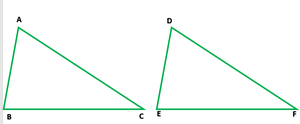Since, △ABC ∼ △DEF,

So,

ar.(△ACB)/ar.(△DEF) = (BC)2/(EF)2

16/25 = (2.3)2/EF2

(4)2/(5)2 = (2.3)2/EF

4/5 = 2.3/EF

EF = (2.3 × 5)/4

EF = 11.5/4 = 2.875 cm

Hence, EF = 2.875 cm

### (ii) If area (△ABC) = 9cm2, area (△DEF) = 64 cm2 and DE = 5.1 cm, find AB.

Solution:

Since, △ABC ∼ △DEF

So,

ar.(△ACB)/ar.(△DEF) = (AB)2/(DE)2

9/5 = AB2/(5.1)2

(3)2/(8)2 = AB2/(5.1)2

3/8 = AB/5.1

AB = (3 × 5.1)/8 = 15.3/8

Hence, AB = 1.9125 cm

### (iii) If AC = 19cm and DF = 8 cm, find the ratio of the area of two triangles.

Solution:

Since, △ABC ∼ △DEF

So,

ar.(△ACB)/ar.(△DEF) = (AB)2/(DE)2

= (19)/(8)2 = 361/64

Hence, ar.(△ABC):ar.(△DEF) = 361:64

### (iv) If area (△ABC) = 36cm2, area (△DEF) = 64 cm2 and DE = 6.2 cm, find AB.

Solution:

Since, △ABC ∼ △DEF

So,

ar.(△ACB)/ar.(△DEF) = (AB)2/(DE)2

⇒ 36/64 = AB2/(6.2)2

⇒ 6/8 = AB/(6.2)

⇒ AB/6.2 = 6/8

⇒ AB = (6 × 6.2)/8 = 37.2/8

AB = 4.65

Hence AB = 4.65 cm

### (v) If AB = 1.2 cm and DE = 1.4 cm, find the ratio of the areas of △ABC and △DEF.

Solution:

Since, △ABC ∼ △DEF

So,

ar.(△ACB)/ar.(△DEF) = (AB)2/(DE)2

(1.2)2/(1.4)2 = 1.44/1.96 = 144/196 = 36/49

Hence, ar.(△ABC):ar.(△DEF) = 36:49

### Question 2. In fig. below ∆ACB ~ ∆APQ. If BC = 10 cm, PQ = 5 cm, BA = 6.5 cm and AP = 2.8 cm, find CA and AQ. Also, find the area (∆ACB): area (∆APQ).

Solution: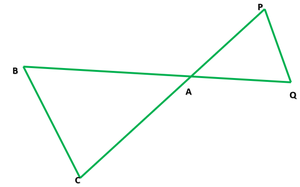According to the question

It is given that, BC = 10 cm, PQ = 5 cm, BA = 6.5 cm and AP = 2.8 cm

Also, △ACB ∼ △APQ

So, BC/PQ = AB/AQ = AC/AP

10/5 = 6.5/AQ = AC/2.8

6.5/AQ = 10/5

⇒ AQ = (6.5 × 5)/10 = 3.25

and AC/2.8 = 10/5

⇒ (2.8 × 10)/5 = 5.6

AC = 5.6m, AQ = 3.25cm

As we know that △ACB ∼ △APQ,

So, ar.(△ACB)/ar.(△APQ) = (BC)2/(PQ)2

ar.(△ACB)/ar.(△APQ) = (10)2/(5)2 = 100/25 = 4/1

Hence, ar(△ACB):ar(△APQ) = 4:1

### Question 3. The areas of two similar triangles are 81 cm2 and 49 cm2 respectively. Find the ratio of their corresponding heights. What is the ratio of their corresponding medians?

Solution:

Let us consider two similar triangles, ABC and PQR whose altitudes are AD and PO

It is given that the areas of two similar triangles are 81 cm2 and 49 cm2

So, △ABC = 81 cm2  and △PQR = 49 cm2

So, ar.(△ABC)/ar.(△PQR) = (AB)2/(PQ)2

81/49 = (AB/PQ)2

9/7 = AB/PQ

Now, in △ABD and △PQO

∠B = ∠Q

Hence, △ABD ~ △PQO

Or

As we know, that the ratio of the areas of two similar triangles are proportional to the square of their corresponding altitudes and also the squares of their corresponding medians.

So, the ratio in their medians = 9 : 7

### Question 4. The areas of two similar triangles are 169 cm2 and 121 cm2 respectively. If the longest side of the larger triangle is 26 cm, find the longest side of the smaller triangle.

Solution:

Given that both the triangles are similar

So, the area of larger triangle(ABC) = 169 cm2

and area of the smaller triangle(PQR) = 121 cm2

The length of the longest sides of the larger triangles(AC) = 26 cm

Let us assume the length of longest side of the smaller triangle(PR) = x

So, the ar.(△ABC)/ar.(△PQR) = (AC)2/(PR)2

169/121 = (26)2/(x)2

13/11 = 26/x

x = (13 × 26)/11

x = 22

Hence, the length of the longest side of the smaller triangle is 22 cm.

### Question 5. The areas of two similar triangles are 25 cm2 and 36 cm2 respectively. If the altitude of the first triangle is 2.4 cm, find the corresponding altitude of the other.

Solution:

Given that, the area of the first triangle = 25 cm2

and the area of second = 36 cm2

Altitude of the first triangle = 2.4 cm

Let us consider the altitude of the second triangle = x

It is given that both the triangles are similar, so

ar.(first triangle)/ar.(second triangle) = (Altitude of the first triangle)2/(Altitude of the second triangle)2

⇒ 25/36 = (2.4)2/x2

⇒ 2.4/x = 5/6

⇒ x = (2.4 × 6)/5 = 14.4/5 = 2.88

Hence, the altitude of the second triangle is 2.88cm

### Question 6. The corresponding altitudes of two similar triangles are 6 cm and 9 cm respectively. Find the ratio of their areas.

Solution:

Given that the length of the corresponding altitude of two triangles are 6 cm and 9 cm

Also, both the triangles are similar

So,

ar.(first triangle)/ar.(second triangle) = (6)2/(9)2

= 36/81

= 4/9

Hence, the ratio of the areas of triangles is 4:9

### Question 7. ABC is a triangle in which ∠A =90°, AN⊥ BC, BC = 12 cm and AC = 5cm. Find the ratio of the areas of ∆ANC and ∆ABC.

Solution: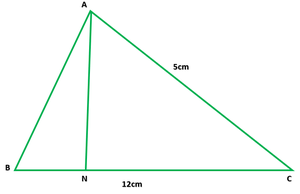Given that,

In ∆ABC, ∠A = 90°

AN ⊥ BC

BC = 12 cm, AC = 5 cm

So, in ∆ANC and ∆ ABC,

∠ANC = ∠BAC = 90°

∠C =∠C              [Common]

So, by AA,

∆ANC ∼ ∆ ABC

ar.(∆ANC)/ar.(∆ABC) = (AC)2/(BC)2 = (5)2/(12)2 = 25/144

Hence, the ratio of the areas of ∆ANC and ∆ABC is 25:144

### Question 8. In Fig., DE || BC

(i) If DE = 4 cm, BC = 6 cm and Area (∆ADE) = 16 cm2, find the area of ∆ABC.

(ii) If DE = 4cm, BC = 8 cm and Area (∆ADE) = 25 cm2, find the area of ∆ABC.

(iii)If DE : BC = 3 : 5. Calculate the ratio of the areas of ∆ADE and the trapezium BCED.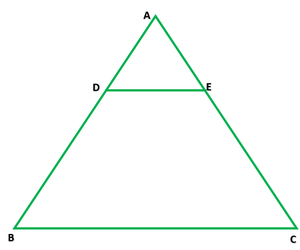Solution:

Given that, DE || BC

∠BAC = ∠DAE

So, by AA

(i) Given that DE = 4 cm, BC = 6 cm and ar(∆ADE) = 16 cm2

As we know that ∆ADE ∼ ∆ABC

16/ar(∆ABC) = 42/62 = 16/36

So,  16 × area ∆ABC = 16 × 36

⇒ ar.(∆ABC) = 36cm2

(ii) Given that DE = 4cm, BC = 8 cm and ar(∆ADE) = 25 cm2

As we know that ∆ADE ∼ ∆ABC

25/area(∆ABC) = (4)2/(8)2 = 16/64

area(∆ABC) = (25 × 64)/16 = 100 cm2

(iii) Given that, DE : BC = 3 : 5

As we know that ∆ADE ∼ ∆ABC

area(∆ADE)/area(∆ABC) = DE2/BC2 = (3/5)2 = 9/25

25 (area(∆ADE)) = 9 (area ∆ABC)

area(∆ADE)/area of trapezium BCED = 9/16

Hence, the ratio of the areas of ∆ADE and the trapezium BCED is 9:16

### Question 9. In ∆ABC, D and E are the mid-points of AB and AC respectively. Find the ratio of the areas of ∆ADE and ∆ABC.

Solution: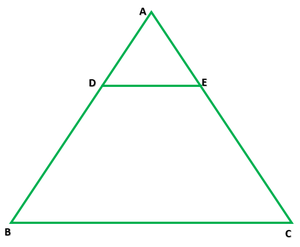Given that, in ∆ABC, D and E are the mid points of AB and AC

So, DE||BC and DE = 1/2BC

∠DAE = ∠BAC   [Common]

By AA

So, ar(∆ADE)/ar(∆ABC) = (DE)2/(BC)2Hence, the ratio of the areas of ∆ADE and ∆ABC is 1:4

### Question 10. The areas of two similar triangles are 100 cm2 and 49 cm2 respectively. If the altitude of the bigger triangle is 5 cm, find the corresponding altitude of the other.

Solution: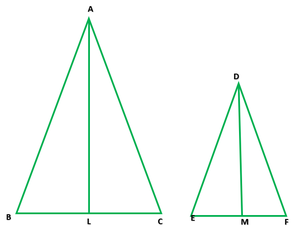Let us consider ∆ABC and ∆DEF

It is given that, area ∆ABC = 100 cm2

and area ∆DEF = 49 cm2

AL perpendicular BC  and DM/EF

AL = 5cm,

Let DM = x cm

It is given that ∆ABC ~ ∆DEF

So, ar(∆ABC)/ar(∆DEF) = AL2/DM2

100/49 = (5)2/(x)2

100/49 = 25/x2

x2 = (25 × 49)/100 = 49/4

x =Hence, the length of altitude of second triangle is 3.5cm

My Personal Notes arrow_drop_up